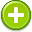#magnitude 中文解釋 wordnet sense Collocation Usage
Noun
/ˈmagnəˌto͞od/,Font size:magnitudes, plural;
1. The great size or extent of something
• - they may feel discouraged at the magnitude of the task before them
2. Great importance
• - events of tragic magnitude
3. Size
• - electorates of less than average magnitude
4. A numerical quantity or value
• - the magnitudes of all the economic variables could be determined
5. The degree of brightness of a star. The magnitude of an astronomical object is now reckoned as the negative logarithm of the brightness; a decrease of one magnitude represents an increase in brightness of 2.512 times. A star with an apparent magnitude of six is barely visible to the naked eye

6. The class into which a star falls by virtue of its brightness

7. A difference of one on a scale of brightness, treated as a unit of measurement

1. the property of relative size or extent (whether large or small); "they tried to predict the magnitude of the explosion"; "about the magnitude of a small pea"
2. order of magnitude: a number assigned to the ratio of two quantities; two quantities are of the same order of magnitude if one is less than 10 times as large as the other; the number of magnitudes that the quantities differ is specified to within a power of 10
3. relative importance; "a problem of the first magnitude"
4. In astronomy, magnitude refers to the logarithmic measure of the brightness of an object, measured in a specific wavelength or passband, usually in optical or near-infrared wavelengths.
5. The magnitude of a mathematical object is its size: a property by which it can be compared as larger or smaller than other objects of the same kind; in technical terms, an ordering (or ranking) of the class of objects to which it belongs. ...
6. In linear algebra, functional analysis and related areas of mathematics, a norm is a function that assigns a strictly positive length or size to all vectors in a vector space, other than the zero vector. A seminorm, on the other hand, is allowed to assign zero length to some non-zero vectors.
7. The absolute or relative size, extent or importance of something; A number, assigned to something, such that it may be compared to others numerically; Of a vector, the norm, most commonly, the two-norm; The apparent brightness of a star (on a negative, logarithmic scale); apparent magnitude; ...
8. (Magnitudes) e.g., the number of planets, type ttw
9. the magnitudes of the various dimensions that are perceived in Phase 2 and Phase 4 of Basic and Enhanced SRV.
10. A scalar value having physical units.
11. Normally refers to the apparent or visual magnitude. It is a measure of the apparent brightness of a celestial object as seen with the naked eye. The greater the brightness, the lower is the value of the magnitude. For example, a star of magnitude +1 is brighter than a star of magnitude +3. ...
12. A measure of the total amount of energy released by an earthquake.
13. A rating of a given earthquake, independent of the place of observation. It is calculated from measurements on seismographs and it is expressed in ordinary numbers and decimals based on a logarithmic scale. ...
14. A general term for objects that have a size and can be ranked.
15. is how much of a pollutant (or pollutant parameter such as toxicity), expressed as a concentration or toxic unit is allowable (USEPA, 1991a.)
16. A number assigned to the properties of an event such that the event can be compared with other events of the same class.
17. The Roman goddess Maia (she who is great) from whom we get the name for the month of May, is also the root of magnitude, and many other words that relate to greatness or size. The greatest value in a set, the Maximum is drawn from the same root. ...
18. A number representing the intrinsic or apparent brightness of a celestial body on a logarithmic scale, in which a difference of one unit corresponds to the multiplication or division of the brightness of light by 2.512.
19. A number that characterizes the relative size of an earthquake. Magnitude is based on measurement of the maximum motion recorded by a seismograph. ...
20. An index of brightness of the stars, with the higher numbers being fainter. Each unit of magnitude increase roughly corresponds to a 2.5 times fall in brightness. The faintest visible stars are 6.0, and rarely with very dark skys and ideal conditions 6.5. ...
21. The numerical size or measure of an attribute (e.g., the area of a polygon, the volume of a solid).
22. A numerical value indicating the brightness of an object in space.
23. The amount of light a star emits or gives off is called its magnitude. Astronomers measure the magnitude of the stars out in the universe by using a number system.
24. Size of the evoked potential generated by the brain.
25. The extent or numeric value of a property